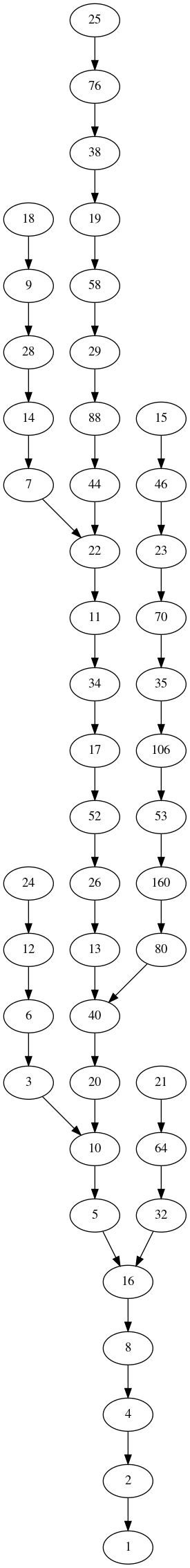# Simple yet mistifying mathematics: the Collatz Conjecture

The Collatz conjecture is arguably the easiest to understand unsolved problem in mathematics. It was famously said that “Mathematics may not be ready for such problems”, and yet the problem itself can be understood by anyone that understands simple arithmetic.

### The problem

We start by picking any whole number. Here we’ll start with 17, but could pick any number. We then generate a new number by applying the following simple rules:

• If our number is even, divide it by 2.
• If it’s odd, multiply it by 3 and then add 1.

So, starting from 17 (an odd number) we reach 52, because we multiply it by 3 as the rule says, giving 51, and then we add 1, giving 52. So 52 is our new number, and we apply that same rule to it - it’s even, so we divide by 2, giving 26.

Our rules have made this sequence:

``````17 -> 52 -> 26
``````

After applying the rules a few more times, we end up with this chain, which ends in 1:

``````17 -> 52 -> 26 -> 13 -> 40 -> 20 -> 10 -> 5 -> 16 -> 8 -> 4 -> 2 -> 1
``````

Now something interesting happens. From 1, we go to 4, as 1 is odd and (3 x 1) + 1 = 4.

``````... -> 1 -> 4
``````

Then, because 4 is even, we divide by 2 and get 2 as our next number:

``````... -> 1 -> 4 -> 2
``````

And 2 is even, so we divide it by 2 and get 1:

``````... -> 1 -> 4 -> 2 -> 1
``````

And we keep going:

``````... -> 1 -> 4 -> 2 -> 1 -> 4 -> 2 -> 1 -> 4 -> 2 -> 1 -> ...
``````

We’re stuck in a loop! The rules force us into this loop of 4 to 2 to 1, and back to 4 again. We can draw this as a chain:

``````17 -> 52 -> 26 -> 13 -> 40 -> 20 -> 10 -> 5 -> 16 -> 8 -> 4 -> 2 -> 1
'----<----'
``````

Going back to our rules, let’s try again, starting from 6. It’s even - divide by two - we get 3. Carry on that chain a few steps, and you’ll see it also gets to 4, then 2, then 1, and gets stuck in the same loop:

``````6 -> 3 -> 10 -> 5 -> 16 -> 8 -> 4 -> 2 -> 1
'----<----'
``````

And if we start a sequence at 53:

``````53 -> 160 -> 80 -> 40 -> 20 -> 10 -> 5 -> 16 -> 8 -> 4 -> 2 -> 1
'----<----'
``````

So it seems like all the starting numbers we try will end in the 4-2-1 loop. Indeed, every starting number everyone has ever tried ends in 4-2-1. Some clever people have written computer programs that check every number, and those programs have tried with extraordinarily large numbers, and all the chains end in 4-2-1.

It turns out that for all our mighty human acheivements - putting men on the moon and inventing computers that send information around the world in less than a second - nobody understands why all the numbers we try end in the 4-2-1 loop. And some of the smartest people to ever live have tried to understand, without success.

The Collatz Conjecture is this unanswered question - do all chains eventually end up at 4-2-1?

This is one of the reasons mathematics is so beautiful to me - we just play a simple little number game that a clever 5 year-old can play, and suddenly we find ourselves at a challenge that is apparently beyond the best and brightest mathematicians to ever live.

### Connecting chains

Going back to our chains, some of the chains we wrote down had parts in common. We can combine the common parts of the chain to create a funny looking network:

``````     53 -> 160 -> 80 ->-.
17 -> 52 -> 26 -> 13 -> 40 -> 20 -> 10 -> 5 -> 16 -> 8 -> 4 -> 2 -> 1
6 -> 3 ->-'                     '----<----'
``````

I wrote a computer program that can draw these networks. If you’re into programming, you can look at my code here. It generated this image, which shows the chain including every number up to 26. I think it’s pretty. Note that it includes some numbers greater than 26, because the rules sometimes generate them - you can see how that happens when we start from 18, 25 or 15.To see the (very cool looking) graph for all numbers up to 1000, click here. The file is quite large and may load slowly, so be patient.